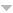## Publications

Export 10 results:
Sort by: Author [ Title] Type Year
O
Fernandes, Vítor H., and Teresa M. Quinteiro. "On the ranks of certain monoids of transformations that preserve a uniform partition." Communications in Algebra. 42.2 (2014): 615-636.
Fernandes, Vítor H., and Jintana Sanwong. "On the rank of semigroups of transformations on a finite set with restricted range." Algebra Colloquium. 21.3 (2014): 497-510.
Fernandes, Vítor H., and Teresa M. Quinteiro. "On the monoids of transformations that preserve the order and a uniform partition." Communications in Algebra. 39.8 (2011): 2798-2815.
Delgado, Manuel, V{\'ı}tor H. Fernandes, Stuart Margolis, and Benjamin Steinberg. "On semigroups whose idempotent-generated subsemigroup is aperiodic." Internat. J. Algebra Comput.. 14 (2004): 655-665.Website
Fernandes, Vítor H., Preeyanuch Honyam, Teresa M. Quinteiro, and Boorapa Singha. "On semigroups of orientation-preserving transformations with restricted range." Communications in Algebra (DOI:10.1080/00927872.2014.975345). 44.1 (2016): 253-264. AbstractWebsite

Let $X_n$ be a chain with n elements ($n\in\N$) and let $\OP_n$ be the monoid of all orientation-preserving transformations of $X_n$. In this paper, for any nonempty subset $Y$ of $X_n$, we consider the subsemigroup $\OP_n(Y)$ of $\OP_n$ of all transformations with range contained in $Y$: we describe the largest regular subsemigroup of $\OP_n(Y)$, which actually coincides with its subset of all regular elements, and Green's relations on $\OP_n(Y)$. Also, we determine when two semigroups of the type $\OP_n(Y)$ are isomorphic and calculate their ranks. Moreover, a parallel study is presented for the correspondent subsemigroups of the monoid $\OR_n$ of all either orientation-preserving or orientation-reversing transformations of $X_n$.

Fernandes, Vítor H., Preeyanuch Honyam, Teresa M. Quinteiro, and Boorapa Singha. "On semigroups of endomorphisms of a chain with restricted range." Semigroup Forum (DOI: 10.1007/s00233-013-9548-x). 89.1 (2014): 77-104. AbstractWebsite

Let $X$ be a finite or infinite chain and let $\O(X)$ be the monoid of all endomorphisms of $X$.
In this paper, we describe the largest regular subsemigroup of $\O(X)$ and Green's relations on $\O(X)$.
In fact, more generally, if $Y$ is a nonempty subset of $X$ and $\O(X,Y)$ is the subsemigroup of $\O(X)$ of all elements with range contained in $Y$,
we characterize the largest regular subsemigroup of $\O(X,Y)$ and Green's relations on $\O(X,Y)$.
Moreover, for finite chains, we determine when two semigroups of the type $\O(X,Y)$ are isomorphic and calculate their ranks.

Fernandes, V. H., M. M. Jesus, and B. Singha. "On orientation-preserving transformations of a chain." Communications in Algebra (DOI 10.1080/00927872.2020.1870996). 49.6 (2021): 2300-2325. AbstractWebsite

In this paper we introduce the notion of an orientation-preserving transformation on an arbitrary chain, as
a natural extension for infinite chains of the well known concept for finite chains introduced in 1998 by McAlister and, independently, in 1999 by Catarino and Higgins.
We consider the monoid POP(X) of all orientation-preserving partial transformations on a finite or infinite chain X and its submonoids OP(X) and POPI(X) of all orientation-preserving full transformations and of all orientation-preserving partial permutations on X, respectively.
The monoid PO(X) of all order-preserving partial transformations on X and its injective counterpart POI(X) are also considered.
We study the regularity and give descriptions of the Green's relations of the monoids POP(X), PO(X), OP(X), POPI(X) and POI(X).

Araújo, Isabel M., Mário J. J. Branco, Vitor H. Fernandes, Gracinda M. S. Gomes, and N. Ruškuc. "On generators and relations for unions of semigroups." Semigroup Forum. 63 (2001): 49-62.
Fernandes, V. H., and M. V. Volkov. "On divisors of semigroups of order-preserving mappings of a finite chain." Semigroup Forum. 81 (2010): 551-554.Website
Fernandes, Vítor H. "On divisors of pseudovarieties generated by some classes of full transformation semigroups." Algebra Colloq.. 15 (2008): 581-588.